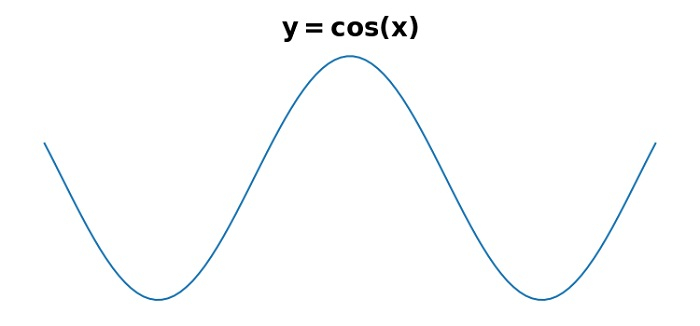# How to use multiple font sizes in one label in Python Matplotlib?

To use multiple font sizes in one label in Python, we can use fontsize in title() method.

## Steps

• Set the figure size and adjust the padding between and around the subplots.

• Create x and y data points using numpy.

• Plot x and y using plot() method.

• Initialize a variable, fontsize.

• Set the title of the plot using title() method with fontsize in the argument.

• Turn off the axes.

• To display the figure, use show() method.

## Example

import numpy as np
from matplotlib import pyplot as plt

plt.rcParams["figure.figsize"] = [7.50, 3.50]
plt.rcParams["figure.autolayout"] = True

x = np.linspace(-5, 5, 100)
y = np.cos(x)

plt.plot(x, y)

fontsize = 20
plt.title("$\bf{y=cos(x)}$", fontsize=fontsize)
plt.axis('off')

plt.show()

## Output